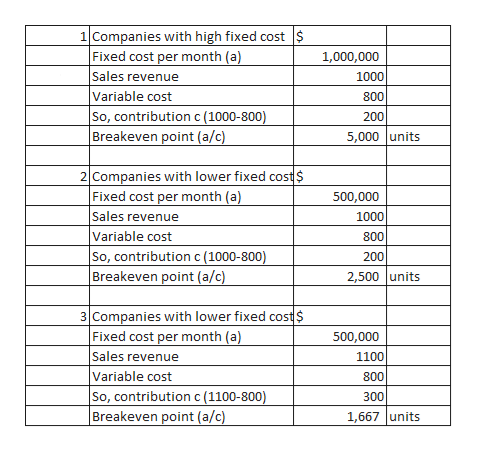Question
4 views

What is breakeven analysis? Give three examples?

check_circle

Step 1

Breakeven analysis is an analysis method to derive the point of sales where the contribution generated on sale is just equal to the fixed costs i.e. at the level of sales where the profit generated is zero. The contribution is computed by subtracting the variable cost from the selling price

Breakeven is computed by following formula

Breakeven point in units= Fixed cost/(Selling price per unit-Variable cost per unit

Step 2

Examples of breakeven analysis ar...help_outlineImage Transcriptionclose1 Companies with high fixed cost \$ Fixed cost per month (a) Sales revenue Variable cost So, contribution c (1000-800) Breakeven point (a/c) 1,000,000 1000 800 200 5,000 units 2 Companies with lower fixed cost\$ Fixed cost per month (a) Sales revenue Variable cost So, contribution c (1000-800) Breakeven point (a/c) 500,000 1000 800 200 2,500 Junits 3 Companies with lower fixed cost\$ Fixed cost per month (a) Sales revenue Variable cost So, contribution c (1100-800) Breakeven point (a/c) 500,000 1100 800 300 1,667 units fullscreen

### Want to see the full answer?

See Solution

#### Want to see this answer and more?

Solutions are written by subject experts who are available 24/7. Questions are typically answered within 1 hour.*

See Solution
*Response times may vary by subject and question.
Tagged in